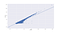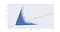# Building a Big Data Machine Learning Spark Application for Flight Delay Prediction

In this tutorial, I explain how to develop a Spark application that allows creating a machine learning model for a real-world problem, using real-world data: Predicting the arrival delay of commercial flights.

To find the best model, several machine learning techniques were used and provided with several inputs combinations. The machine learning methods explored are Linear Regression, Random Forest Trees and Gradient-Boosted Trees. All the code will be written in Scala.

The full code for this application and how to run it can be found in the GitHub repo: https://github.com/pedroduartecosta/Spark-PredictFlightDelay

# Problem

We want to be able to predict, based on historical data, the arrival delay of a flight using only information available before the flight takes off. Since the target variable (ArrDelay - arrival delay) is a numerical value we will use regression algorithms only, I’ll explain this further below.

# Dataset

The data used was published by the US Department of Transportation and can be downloaded here. It compromises almost 23 years worth of data, and analysing such amount of data requires big data tools such as Spark.

# Setting up the project

For those who are new to Spark, I provide a tutorial on how to set up the project and its dependencies using SBT.

The tutorial can be found here: https://medium.com/@pedrodc/setting-up-a-spark-machine-learning-project-with-scala-sbt-and-mllib-831c329907ea

# Exploratory Data Analysis

The most important part of a data science project is to perform a good analysis of the problem and the data we have. Since most variables are numerical we will first create a correlation matrix, of the numerical variables, to understand if we can find any correlation between them.

## Correlation explained

A correlation describes the strength of association between two attributes. The closer the value is to 1 or -1, the more positively or negatively the variables are correlated. A correlation of zero means that that the two attributes are not correlated at all. A coefficient of +1 indicates that the two attributes have a perfect correlation, meaning every change in one attribute is accompanied by an equivalent change in the other attribute in the same direction. A coefficient of -1 indicates that every change in one attribute is accompanied by the opposite change in the other attribute. —based on the book Data Science by MIT Press

Looking at the correlation matrix we can see that the DepDelay (departure delay) it highly correlated to arrival delay, so the departure delay might be the most indicative attribute of a flight delay. Looking a bit further we can also see a weak, but existing correlation, between the TaxiOut and arrival delay. Therefore we will be looking further into these two variables.Scatterplot between departure delay and arrival delay from the 2008 dataset.Scatterplot between taxi-out duration and arrival delay from the 2008 dataset.

These two plots allow us to better understand the correlation value presented before.

# Implementation

Any implementation mentioned and not presented in code here, it is because the code should be trivial and can be found in full in the repository.

So first we read the data from the CSV file using the spark SQLContext. To load multiple CSV files, simply provide a path such as: /mydata/*.csv. This will load the data into a DataFrame which is a distributed collection of data organized into named columns. So if we are running the spark application in a cluster this will distribute the data randomly across the cluster.

Then we use the .drop() function to drop all the variables that are only known after the flight took off and also variables that are too correlated between themselves and can be redundant to the model may be causing the model to overfit. We also drop cancelled flights and variables that are not correlated to the arrival delay.

## Processing the data

The data processing highly depends on the machine learning algorithm implementation and on the experiment being done. The main difference will be in what variables are dropped and if we use nominal (categorical) values, such as, the Airport of origin and destination.

To use the categorical variables in regression algorithms, further processing was needed. These variables were converted to numerical values by applying a StringIndexer and OneHotEncoder. More information on these two methods and why there were used can be found on the links.

## Training the data and obtaining a model

We found attributes that demonstrate a high linear correlation, departure delay with arrival delay, which can be seen in the first scatterplot. Therefore the first method we will test on is Linear Regression. This is a very fast and simple algorithm which provides good results in these circumstances.

Here we create a pipeline to process all the transformations on the data. This allows us to define all the steps and only run them when the function .fit() is called to train the model. The pipeline compromises the following steps: converting the categorical values (if it applies) to numerical ones, then assembling all in a features vector and running the machine learning algorithm to obtain a model.

The code for running Random Forest Trees and Gradient-Boosted Trees machine learning algorithms can be found in the repository and these methods integrate seamlessly in this pipeline. They base their implementation on decision trees and require much more space and time to run but might provide better results when the features and target variable relation is not linear. More information can be found here.

Before the data is used, it must first be split in training and testing sets, a split of 70% was used, in order to later evaluate the resulting model.

The golden rule for evaluating models is that models should never be tested on the same data they were trained on. — Data Science, MIT Press

## Optimizations

We use the TrainValidationSplit method to split the training data in training and validating data. The validating data is used to optimize the algorithm parameters. A ParamGridBuilder is used to define the parameters range in which the machine learning algorithm will be trained on. The best performing set of parameters will be then used to obtain the final model.

## Evaluating the resulting model

Two main metrics were used to evaluate the model’s performance. R squared and Root Mean Square Error(RMSE).

RMSE, measures the difference between values predicted by a model and the values observed. Considering this, the lower RMSE value is, the better is the model.

R Squared, also known as the coefficient of determination, it is a statistical measure of how close the data is to the fitted regression line. It assesses the goodness of fit of our regression model. The closer the value is to 1, the better is the model.

# Final considerations

In the tests performed, the Linear Regression algorithm performed better than the other two algorithms based on decision trees. This can be due to the linear relation of the features to the target variable used. The results and further analysis can be found in the report in the repository.

Spark is a very powerful tool, the code and application here demonstrated can run on a cluster without any alteration, allowing to test huge datasets of data with more complex algorithms.

Feel free to reach me if you have any questions: me@pedrocosta.eu

Written by

Written by

## Pedro Costa

#### Full Stack Engineer | AWS Certified Solutions Architect — Associate

Welcome to a place where words matter. On Medium, smart voices and original ideas take center stage - with no ads in sight. Watch
Follow all the topics you care about, and we’ll deliver the best stories for you to your homepage and inbox. Explore
Get unlimited access to the best stories on Medium — and support writers while you’re at it. Just \$5/month. Upgrade